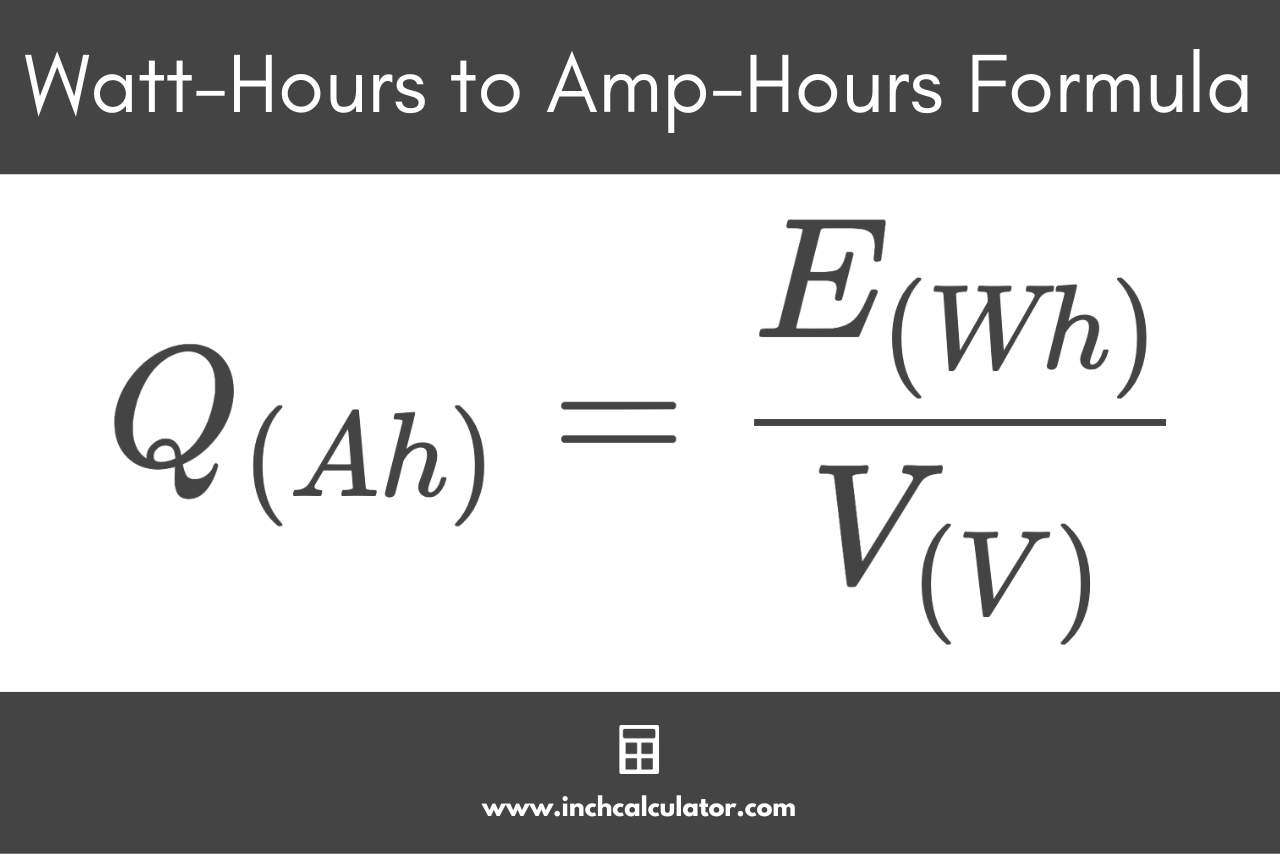# Watt-Hours (Wh) to Amp-Hours (Ah) Conversion Calculator

Convert watt-hours to amp-hours by entering the energy in watt-hours and the battery terminal voltage below.

Wh
V

## Amp-Hours Results:

Ah
Learn how we calculated this below

## How to Convert Watt-Hours to Amp-Hours

Watt-hours (Wh) and ampere-hours (Ah) are units that are often used to measure battery capacity.

The watt-hour capacity of a battery, expressed as Wh or W·h, is a measure of the amount of energy being supplied to the load.

The ampere-hour capacity of a battery, expressed as Ah or A·h, describes the duration for which a battery can supply one ampere of current and the maximum amount of current it can supply for one hour.

To convert watt-hours to amp-hours, you can use the Ohm’s Law formula.

### Wh to Ah Conversion Formula

The formula to convert energy to electrical charge using Ohm’s Law is:

Q(Ah) = E(Wh) / V(V)

Thus, the charge in amp-hours is equal to the watt-hours divided by the voltage. This is essentially the same formula used to convert watts to amps.You can also convert Ah to Wh using a similar formula.

For example, let’s convert 600 Wh at 12 V to Ah.

Q(Ah) = 600 Wh / 12 V
Q(Ah) = 50 Ah

So, 600 watt-hours of energy at 12 volts is equal to a charge of 50 amp-hours.

You might also be interested in our kilowatt-hours to amp-hours calculator.